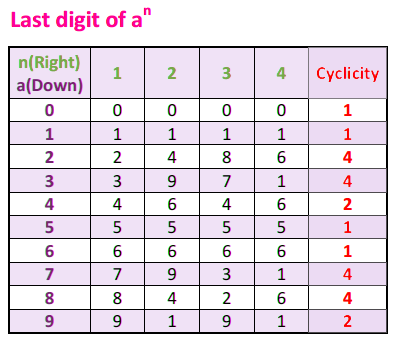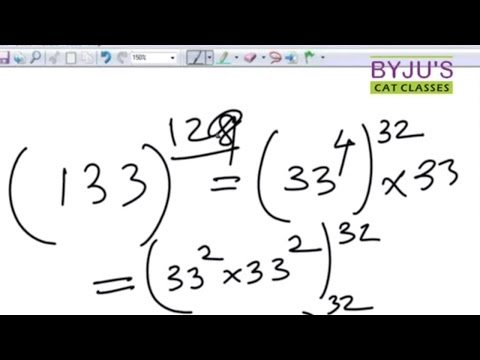# Number Systems Shortcut For CAT Exam

Over the years, CAT examination has the same pattern of questions for its Quantitative Aptitude. Quantitative Aptitude will have problems categorized as:

1. Number System
2. Geometry
3. Modern Maths
4. Algebra
5. Arithmetic

Finding the last two digit comes under the Number System category of quantitative aptitude and after Geometry, the Number system is the part from where most questions are expected.

### Multiplying the last two digit of the exponents in ten seconds.

Follow this trick and shortcut method to multiply the numbers in your head. This can be a huge advantage for those preparing for CAT exam or any other MBA entrance exams.
The tricks used in this video is simple and straight. You might not need a paper and pen to solve this type of question after learning the shortcuts.

The last digit of a number in form: an

The simple trick followed to know the last digit is to remember the cyclicity of the last digit of the number given. Every digit from 1 to 9 follow a pattern when exponential power is applied.  Here is the table that will be handy in solving these type of questions.The following video is the shortcut technique to find the last two digit of any power expression. With this technique, you will be able to solve where the last two digit of the numbers are required to be found out.

Here, an example of the numbers ending in 1, like – 3167, 4123
Later it was discussed on how to calculate to do the last two digit of the expression- 3764

This technique can be used for any number, asking the last digit raised to any number. If you have to solve this type of question, you will be able to solve it within 30 seconds after watching the video.Make your calculation skills phenomenal with these tricks and tips from BYJU’S. Check out the detailed process of registering for CAT exam 2021. Post any CAT related question on BYJU’S Forum or Call our education counselor at 09880031619 and prepare for CAT exam from the best CAT coaching institute in India.

Stay tuned with BYJU’S to get all CAT related details along with various preparation tips for all the important topics. Also, get similar video engaging video lessons to learn various shortcut tricks to solve the questions.